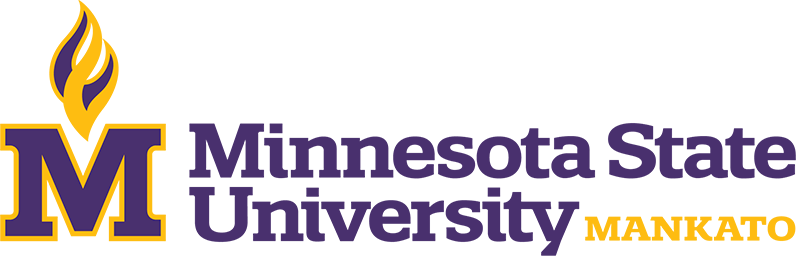Catalog Year

Degree

Credits

Locations

# Statistics Minor## Program Requirements

### Core

Limits, continuity, the derivative and applications, transcendental functions, L'Hopital's Rule, and development of the Riemann integral.

Prerequisites: Satisfy Placement Table in this section, MATH 115 or both MATH 112 and MATH 113 with "C" (2.0) or better.

Goal Areas: GE-04

Techniques of integration, applications of integration, improper integrals, numerical integration, the calculus of parametric curves, and infinite series and sequences.

Prerequisites: MATH 121 with "C" (2.0) or better or consent

A calculus based introduction to probability and statistics. Topics include probability, random variables, probability distributions (discrete and continuous), joint probability distributions (discrete and continuous), statistical inference (both estimation and hypothesis testing), confidence intervals for distribution of parameters and their functions, sample size determinations, analysis of variance, regression, and correlation. This course meets the needs of the practitioner and the person who plans further study in statistics. Same as MATH 354. Prereq: MATH 122 with C or better or consent Fall, Spring, Summer

Prerequisites: MATH 122 with C or better or consent

Simple and multiple linear regression, model adequacy checking and validation, identification of outliers, leverage and influence, polynomial regression, variable selection and model building strategies, nonlinear regression, and generalized linear regression.

Prerequisites: MATH 354 / STAT 354 or STAT 455 with "C" (2.0) or better or consent

Randomized complete block design, Latin squares design, Graco- Latin squares design, balanced incomplete block design, factorial design, fractional factorial design, response surface method, fixed effects and random effects models, nested and split plot design.

Prerequisites: MATH 354 / STAT 354 or STAT 455 with "C" (2.0) or better or consent

### Restricted Electives

Choose 3 - 4 Credit(s).

A mathematical approach to statistics with derivation of theoretical results and of basic techniques used in applications. Includes probability, continuous probability distributions, multivariate distributions, functions of random variables, central limit theorem and statistical inference. Same as MATH 455. Prereq: MATH 223 with C or better or consent

Prerequisites: MATH 223 with "C" (2.0) or better or consent

Sampling distributions: means and variances. Bias, robustness and efficiency. Random sampling, systematic sampling methods including stratified random sampling, cluster sampling and two-stage sampling, ratio, regression, and population size estimation. Suitable statistical software is introduced, for example, MATLAB, R, SAS, etc.

Prerequisites: Either MATH/STAT 354 or both MATH 121 adn STAT 154 with "C" (2.0) or better, or consent.

Forms of multivariate analysis for discrete data, two dimensional tables, models of independence, log linear models, estimation of expected values, model selection, higher dimensional tables, logistic models and incompleteness. Logistic regression. Suitable statistical software is introduced, for example, MATLAB, R, SAS etc.

Prerequisites: Either MATH/STAT 354 or both MATH 121 and STAT 154 with "C" (2.0) or better, or consent.

Derivation and usage of nonparametric statistical methods in univariate, bivariate, and multivariate data. Applications in count, score, and rank data, analysis of variance for ranked data. Nonparametric regression estimation. Suitable statistical software is introduced, for example, MATLAB, R, SAS, etc.

Prerequisites: Either MATH/STAT 354 or both STAT 154 and MATH 121 with "C" (2.0) or better, or consent.